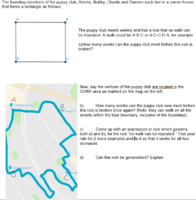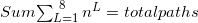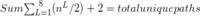Combinatorics Problem with counting unique paths.

Gaz

New member
I'm not sure if this is the right board. Wasn't sure where to post.For question one I've assumed that a subsection repeat where the same order is repeated in one walk, e.g. A-B-A-B (ab is repeated) is restricted.
without this there would be infinite paths i assume.

For question one starting from A as the selected point.
(2^(L)) + (2^(L+1)) + (2^(L+2)) + (2^(L+3)) + (2^(L+4)) + (2^(L+5)) + (2^(L+6)) + (2^(L+7) where L is equal to 1, and the twos in there are basically n where n is equal to the amount of options at each of the vertices (2). The reason i've stopped at 8"(L+7)" is because a 9 edge/sub section walk must contain some repeated walks therefore it is void based on my assumptions. this long string can also be written as

Sum \$\sum_{L=1}^{\8} n^{L} = total pathsbasically this, but then including my subsection restriction rule for each point i have to change n^L by /2+2 to get the non restricted pathways. therefore,which is equal to 271, minus 1 = 270 unique pathways. the one is subtracted,it is including picking just A as a walk, yet physically it would be impossible for the puppy club to walk and start at A, as they wouldn't be walking anywhere.

therefore for all points, not just from A, the total unique paths are 4 * ( my sum formula ).
4 * 270 = 1350
1350 weeks the puppy club could walk without being repeated, inclusive of starting from any of the four points.

For question two, i have no idea how to solve it. would appreciate any help.

Attachments

• 2.1 KB Views: 1

Jomo

Elite Member
Why do you think that the walks has to only include points a, b, c and d? Just because the four members live at those corners does not prohibit the club members from venturing elsewhere. Did you leave out part of the problem??

Gaz

New member
I'm not sure what you mean, which question are you referring to? The first, more simple one with four points or the bottom one?